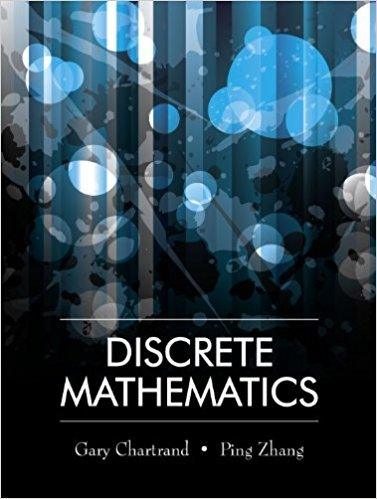×
×

# Solutions for Chapter 9.2: Permutations and Combinations with Repetition## Full solutions for Discrete Mathematics | 1st Edition

ISBN: 9781577667308Solutions for Chapter 9.2: Permutations and Combinations with Repetition

Solutions for Chapter 9.2
4 5 0 372 Reviews
26
2
##### ISBN: 9781577667308

Chapter 9.2: Permutations and Combinations with Repetition includes 26 full step-by-step solutions. Discrete Mathematics was written by and is associated to the ISBN: 9781577667308. This textbook survival guide was created for the textbook: Discrete Mathematics, edition: 1. This expansive textbook survival guide covers the following chapters and their solutions. Since 26 problems in chapter 9.2: Permutations and Combinations with Repetition have been answered, more than 13003 students have viewed full step-by-step solutions from this chapter.

Key Math Terms and definitions covered in this textbook
• Characteristic equation det(A - AI) = O.

The n roots are the eigenvalues of A.

• Cramer's Rule for Ax = b.

B j has b replacing column j of A; x j = det B j I det A

• Fourier matrix F.

Entries Fjk = e21Cijk/n give orthogonal columns FT F = nI. Then y = Fe is the (inverse) Discrete Fourier Transform Y j = L cke21Cijk/n.

• Gauss-Jordan method.

Invert A by row operations on [A I] to reach [I A-I].

• Left inverse A+.

If A has full column rank n, then A+ = (AT A)-I AT has A+ A = In.

• Left nullspace N (AT).

Nullspace of AT = "left nullspace" of A because y T A = OT.

• Pascal matrix

Ps = pascal(n) = the symmetric matrix with binomial entries (i1~;2). Ps = PL Pu all contain Pascal's triangle with det = 1 (see Pascal in the index).

• Polar decomposition A = Q H.

Orthogonal Q times positive (semi)definite H.

• Right inverse A+.

If A has full row rank m, then A+ = AT(AAT)-l has AA+ = 1m.

• Rotation matrix

R = [~ CS ] rotates the plane by () and R- 1 = RT rotates back by -(). Eigenvalues are eiO and e-iO , eigenvectors are (1, ±i). c, s = cos (), sin ().

• Similar matrices A and B.

Every B = M-I AM has the same eigenvalues as A.

• Skew-symmetric matrix K.

The transpose is -K, since Kij = -Kji. Eigenvalues are pure imaginary, eigenvectors are orthogonal, eKt is an orthogonal matrix.

• Spectrum of A = the set of eigenvalues {A I, ... , An}.

Spectral radius = max of IAi I.

• Standard basis for Rn.

Columns of n by n identity matrix (written i ,j ,k in R3).

• Symmetric factorizations A = LDLT and A = QAQT.

Signs in A = signs in D.

• Toeplitz matrix.

Constant down each diagonal = time-invariant (shift-invariant) filter.

• Tridiagonal matrix T: tij = 0 if Ii - j I > 1.

T- 1 has rank 1 above and below diagonal.

• Vandermonde matrix V.

V c = b gives coefficients of p(x) = Co + ... + Cn_IXn- 1 with P(Xi) = bi. Vij = (Xi)j-I and det V = product of (Xk - Xi) for k > i.

v + w = (VI + WI, ... , Vn + Wn ) = diagonal of parallelogram.

• Vector space V.

Set of vectors such that all combinations cv + d w remain within V. Eight required rules are given in Section 3.1 for scalars c, d and vectors v, w.

×